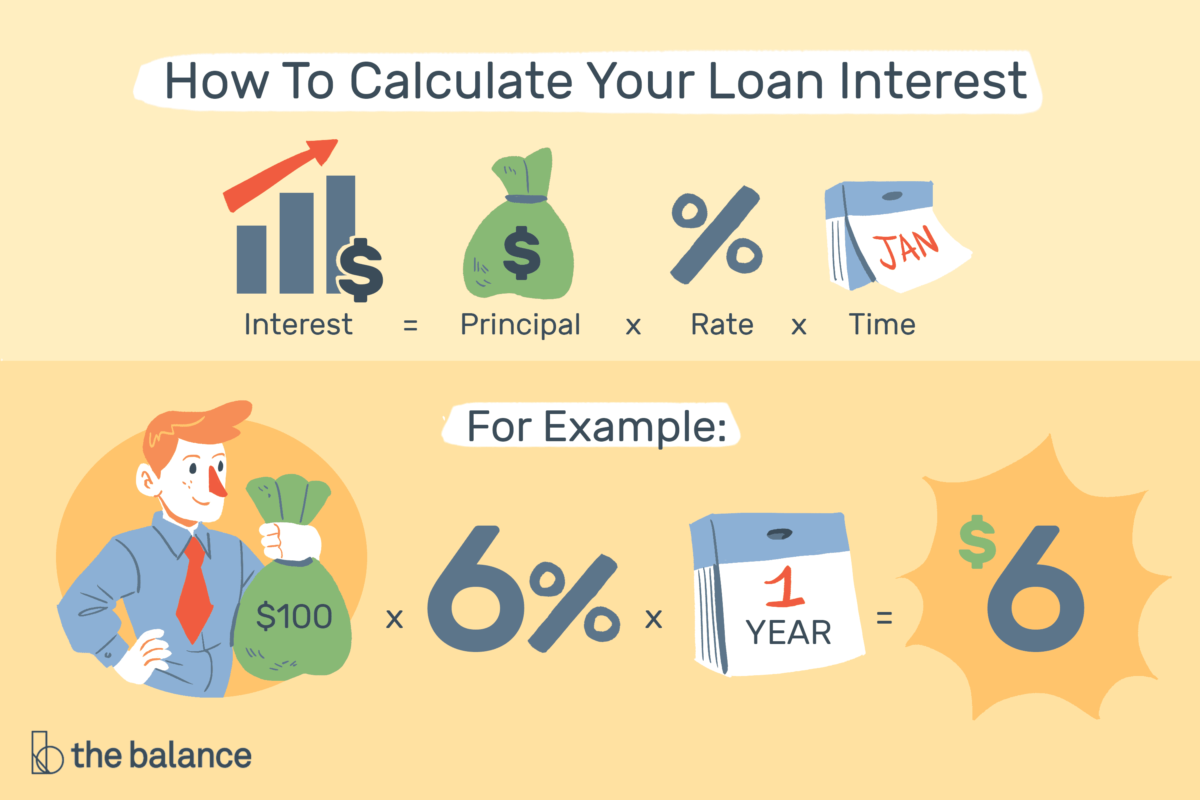# Compute Loan Interest With Calculators or Templates

The easiest way to compute loan interest is with a calculator or spreadsheet, but you can also do it by hand if you prefer. For quick answers, use technology—online calculators or spreadsheets. To understand the details, do a portion of the math yourself. You’ll make more informed decisions when you understand the numbers.

## Types of Interest

To get the correct information, you need to understand exactly how interest is charged, and that depends on the loan in question and the lender’s rules.

For example, credit cards often charge interest daily—so it’s worth it to make a payment as soon as possible. Other lenders might calculate interest monthly or annually. This detail is important because you need to use the right numbers for your calculations. Lenders typically quote interest rates as an annual percentage rate (APR). But if you pay interest monthly, you must convert that rate to a monthly rate by dividing by 12 for your calculations. For example, a 12% annual rate becomes a 1% monthly rate.

If you want to do as little math as possible, there are two ways to take advantage of technology:

• Spreadsheets: Microsoft Excel, Google Sheets, and other programs make it easy to build a model of your loan. With a basic model, you can change inputs to see how different loans compare, and view total lifetime interest costs.
• Loan amortization calculator: This tool will calculate your monthly payment, show how much interest is in each payment, and show how much you pay down your balance every month.

## How to Compute Loan Interest Yourself

If you prefer not to use a spreadsheet or calculator, you can do it all by hand and become a pro at understanding interest expenses.

For standard home, auto, and student loans, the best way to do this is to build an amortization table. This table details every payment, monthly interest, and principal amounts, and your remaining loan balance at any given time (just like a spreadsheet or a good calculator does). To complete a calculation, you’ll need several pieces of information:

• The interest rate
• The length of time the loan lasts
• The loan balance you’re paying interest on (known as the principal)
• The monthly payment

For a quick estimate of interest costs, a simple interest calculation may get you “close enough.”

## Simple Interest Example

Assume you borrow \$100 at 6% for one year. How much interest will you pay?

• Interest = Principal x rate x time
• Interest = \$100 x .06 x 1
• Interest = \$6

Most loans aren’t that simple. You repay over many years, and interest is charged every year, sometimes even compounding and causing your balance to grow. In the case of compounding interest, you accrue interest charges on any unpaid interest.

## Real-Life Example

Assume you borrow \$100,000 at 6% APR to be repaid monthly over 30 years. How much interest will you pay? Assume this is a standard installment loan, like a home loan. (Hint: The monthly payment is \$599.55.)

You’ll actually pay a different amount of interest every month—ideally, the amount decreases each month. These loans go through a process called amortization, which reduces your loan balance over time as you continue to make payments.

The table at the bottom shows how your loan calculations might look. Total interest over the first three payments is \$1,498.50 (\$500 + \$499.50 + \$499). To build that table yourself, use the steps below:

1. Calculate the monthly payment.
2. Convert the annual rate to a monthly rate by dividing by 12 (6% annually divided by 12 months results in a 0.5% monthly rate).
3. Figure the monthly interest by multiplying the monthly rate by the loan balance at the start of the month (\$100,000 multiplied by 0.5% equals \$500 for the first month).
4. Subtract the interest costs from the monthly payment. Keep a running tally in an additional column if you want to track interest over time.
5. Apply the remainder of the monthly payment to principal repayment. This is how you reduce your loan balance—through principal payment.
6. Calculate your remaining loan balance.
7. Copy the remaining loan balance to the beginning of the next line.
8. Repeat steps 2 through 8 until the loan is paid off.

You’ll see that a portion of each payment goes to interest expenses, while the rest pays down the loan balance. Payments in the early years primarily cover your interest costs, and this is especially true for long-term loans like a mortgage. Over time, the interest portion decreases, and you pay down the loan more quickly.

## Calculating Credit Card Interest

With credit cards, the calculation is similar, but it can be more complicated. Your card issuer may use a daily interest method or assess interest monthly based on an average balance, for example. Minimum payments will also vary by card issuer, depending on the card issuer’s approach to generating profits. Check the fine print in the credit card agreement to get the details.

## Interest Expenses

Interest effectively raises the price of the things you buy, whether it’s a new home, a car, or equipment for your business. In some cases, those interest costs are tax-deductible—which is one more reason not to ignore them. In other cases, interest is simply the price you pay for using someone else’s money.

To understand your finances, it’s wise to calculate interest costs any time you borrow. This allows you to compare the costs of different loans and will help you evaluate big decisions such as how much to spend on a house or automobile. You can compare lenders, choose between longer or shorter loan terms, and find out how much the interest rate really affects your total interest costs.

Source: thebalance.com ~ Image: thebalance.com